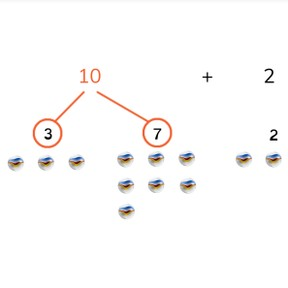8,000 schools use Gynzy92,000 teachers use Gynzy1,600,000 students use Gynzy

## General

Students learn to group numbers to add to 20 using three addends. They learn to make ten, and then to add the third addend.

## Introduction

Students see all kinds of numbers on the interactive whiteboard. They must select two numbers whose total is 10. They can circle these numbers.

## Development

Show students the image. Show that Jose has 3 marbles, Brandon has 7 marbles, and Lynn has 2 marbles. Ask how they can figure out how many marbles the kids have together. Explain that the first step is to see if you can make ten, which two numbers add together to make ten? Then you add the last number. Then show the tennis-ball problem. Search for the two numbers which add to 10 (the first two), and then add the third number. Show this with the next problem, but it is the last two numbers which add to 10 together, so you add those first, and then the third number. Use this to point out to students that it doesn't have to be the first two numbers of the three which add to 10.
Next students practice finding the two numbers that total 10. The students calculate the answers to the problems shown on the board. In the left row, the first two numbers make ten, whereas in the right row, they are mixed up. To finish up, students practice adding the problems presented as story problems.

Check that students understanding adding to 20 making ten with three addends by asking the following questions:
- How do you group numbers?
- What do you pay attention to when choosing addends?
- What do you pay attention to when adding?
- What are the steps you take?
- Do you only pay attention to the first two numbers?

## Guided practice

Students start with problems in which the first two numbers make 10. In the second exercise, the first and last number make 10. Finally students use the numbers from a story problem.

## Teaching tips

Students who have difficulty with the order of addition of three addends can first practice searching for the two addends that make 10.
They can use MAB-blocks or other manipulatives to set out each number. They can also practice decomposing 10.

## Instruction materials

dice and blocks

### The online teaching platform for interactive whiteboards and displays in schools

• Save time building lessons

• Manage the classroom more efficiently

• Increase student engagement